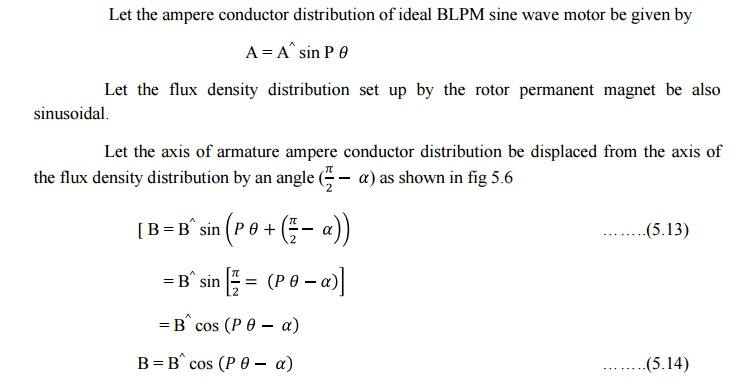Home | | Special Electrical Machines | Torque Equation of BLPM Sine Wave Motor

# Torque Equation of BLPM Sine Wave Motor

1. Ampere conductor density distribution 2. Torque equation of an ideal BLPM sine wave motor 3. Torque developed in a practical BLPM SNW motor

TORQUE EQUATION OF BLPM SINE WAVE MOTOR

1. Ampere conductor density distribution2. Torque equation of an ideal BLPM sine wave motorLet the fig. 5.5 shows the ampere conductor density distribution in the air gap due to the current carrying armature winding be sinusoidal distributed in the airgap space.As the armature conductors are located in stator of the BLPM SNW motor, the rotor experiences an equal and opposite torque.

Torque experienced by the rotor

= Torque developed by the rotorΒ is known as power angle or torque angle. T= π A B rl sin β in an ideal motor.

Consider the case of an armature winding which has three phases. Further the winding consists of short chorded coils and the coils of a phase group are distributed. The 3 phase armature winding carries a balanced 3 phase ac current which are sinusoidally varying. The various phase windings are ph a, ph b and ph c.

The axis of phase winding are displaced by 2π/3p mechanical radians or 2π/3 elec. Radians.

The current in the winding are also balanced. An armature winding is said to be balanced if all the three phase winding are exactly identical in all respects but there axes are mutually displaced by 2π/3p mech radians apart.

A three phase armature current is said to be balanced when the 3 phase currents are exactly equal but mutually displaced in phase by 120 degree.When the 3 phase ac current passes through the 3 phase balanced winding it sets up an armature mmf in the air gap.

Space distribution of the fundamental component of armature ampere conductors can be written as.3. Torque developed in a practical BLPM SNW motor:

v   Ampere turn distribution of a phase winding consisting of full pitched coil is rectangular of amplitude I T ph. But the fundamental component of this distribution is the fundamental component of this distribution is 4/πi Tph.

v   In a practical motor, the armature turns are short chorded and distributed .Further they may be accomonadated in skewed slots. In such a case for getting fundamental component of ampere turns distribution the turns per phase is modified as Kw1 Tph where Kw1 is winding factor which is equal to Ks1 Kp1 Kd1

Ks1 = Skew factorProperties of 'A‘ ( Ampere conductor density);

v   Ampere conductor density is sinusoidally distributed in space with amplitude Â. This distribution has 2p poles (i.e) same as the rotor permanent magnetic field.

v   The ampere conductor distribution revolves in air gap with uniform angular velocity ώm rad /sec .or ώelec.rad/sec.(Ns rpm). This is the same speed as that of rotor magnetic field.

v   The direction of rotation of armature ampere conductor distribution is same as that of rotor. This is achieved by suitably triggering the electronic circuit from the signals obtained from rotor position sensor.

v   4. The relative angular velocity between sine distributed permanent magnetic field and sine distributed armature ampere conductor density field is 0. Under such condition it has been

shown an electromagnetic torque is developed whose magnitude is proportional to sin β. β-torque angle or power angle.

Angle between the axes of the two fields is π/2-α and β=-α Torque developed by the motor = 3EphIphsinβ/ώmN-m

ώm=2πNs/60        where NS is in rpm

T=60/2πNs (3EphIphsinβ)

=3EphIphsinβ syn.watts. 1 syn.watt=60/2πNs N-m

It is a machine dependent conversion factor

Study Material, Lecturing Notes, Assignment, Reference, Wiki description explanation, brief detail
Special Electrical Machines : Permanent Magnet Synchronous Motor : Torque Equation of BLPM Sine Wave Motor |